# Rotational Motion

Rigid Body: A rigid body consists of a number of particles such that the distance between any pair of particles always remains constant.

Moment of Inertia (Rotational Inertia) I: -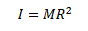1. Mass of body

2. Distribution of mass about the axis of rotation

3. Moment of inertia of a body should always be referred to as about a given axis, since it depends upon distribution of mass about that axis.

4. It does not depend upon the state of motion of rotating body. It is same whether the body is at rest, rotating slowly or rotating fast about the given axis. I=∑mr2

Rotational Kinetic Energy: -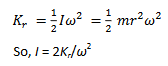Radius Gyration: - Radius of gyration of a body about a given axis is that distance, at which if whole of the mass of the body were concentrated, it would have same moment of inertia as that of body.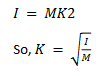Again, Radius of gyration of a body about a given axis is defined as the square root of the mean of the squares of distances of various particles of the body from the axis of rotation.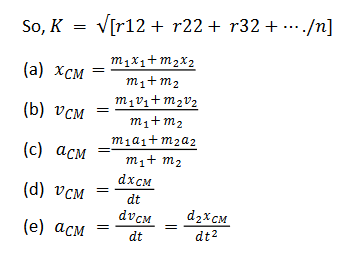System of mass for many particle system: -

1. Perpendicular axes theorem: -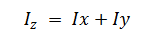2. Parallel axes theorem: -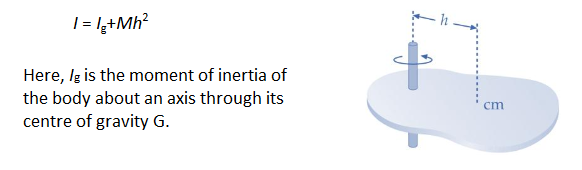Moments of Inertia of Various Objects: -